# 不得不谈的限流算法

## 何为限流？

``````X-RateLimit-Limit: 60         //每秒60次请求
X-RateLimit-Remaining: 23     //当前还剩下多少次
X-RateLimit-Reset: 1540650789 //限制重置时间
``````

## 算法介绍

### 1. 计数器法

1. 可以在程序中设置一个变量 `count`，当过来一个请求我就将这个数 `+1`，同时记录请求时间。
2. 当下一个请求来的时候判断 `count` 的计数值是否超过设定的频次，以及当前请求的时间和第一次请求时间是否在 1 分钟内。
• 如果在 1 分钟内并且超过设定的频次则证明请求过多，后面的请求就拒绝掉。
• 如果该请求与第一个请求的间隔时间大于 1 分钟，且 `count` 值还在限流范围内，就重置 `count`

``````type Counter struct {
Count       uint   // 初始计数器
Limit       uint   // 时间窗口最大请求频次
Interval    int64  // 单位毫秒 ms
RefreshTime int64  // 时间窗口
}

func (c *Counter) rateLimit() bool {
now := time.Now().Unix()
if now < (c.RefreshTime + c.Interval) {
// 在时间窗口内
c.Count++
return c.Count <= c.Limit
} else {
c.RefreshTime = now
c.Count = 0 // 超时重置
return true
}
}
``````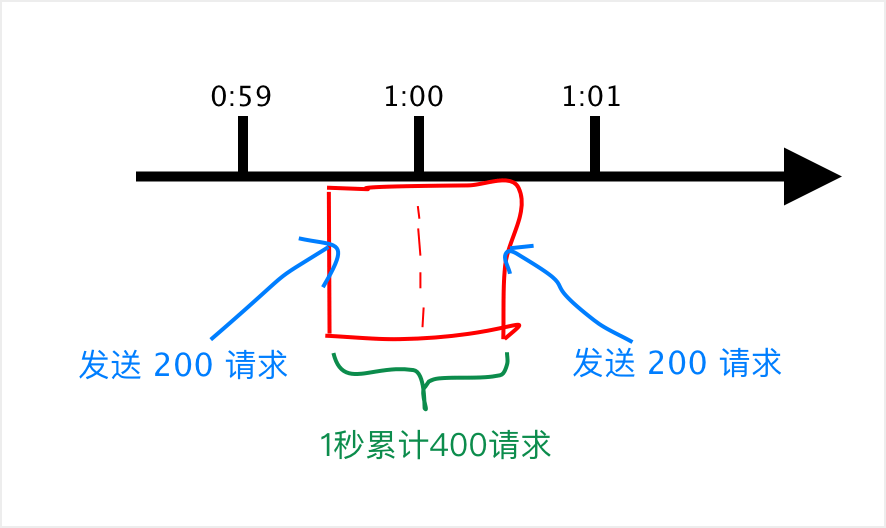### 2. 滑动窗口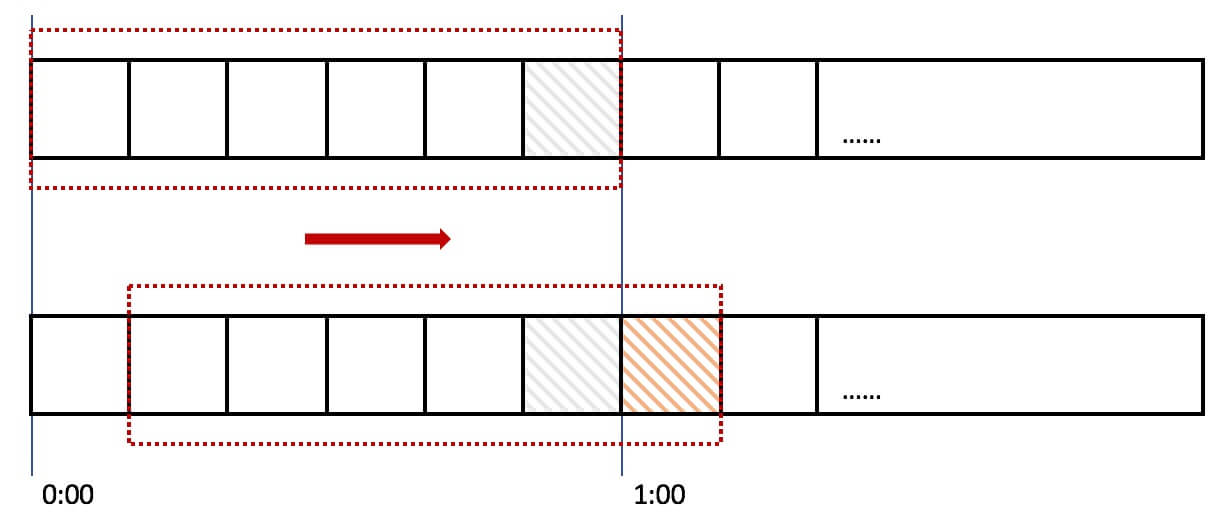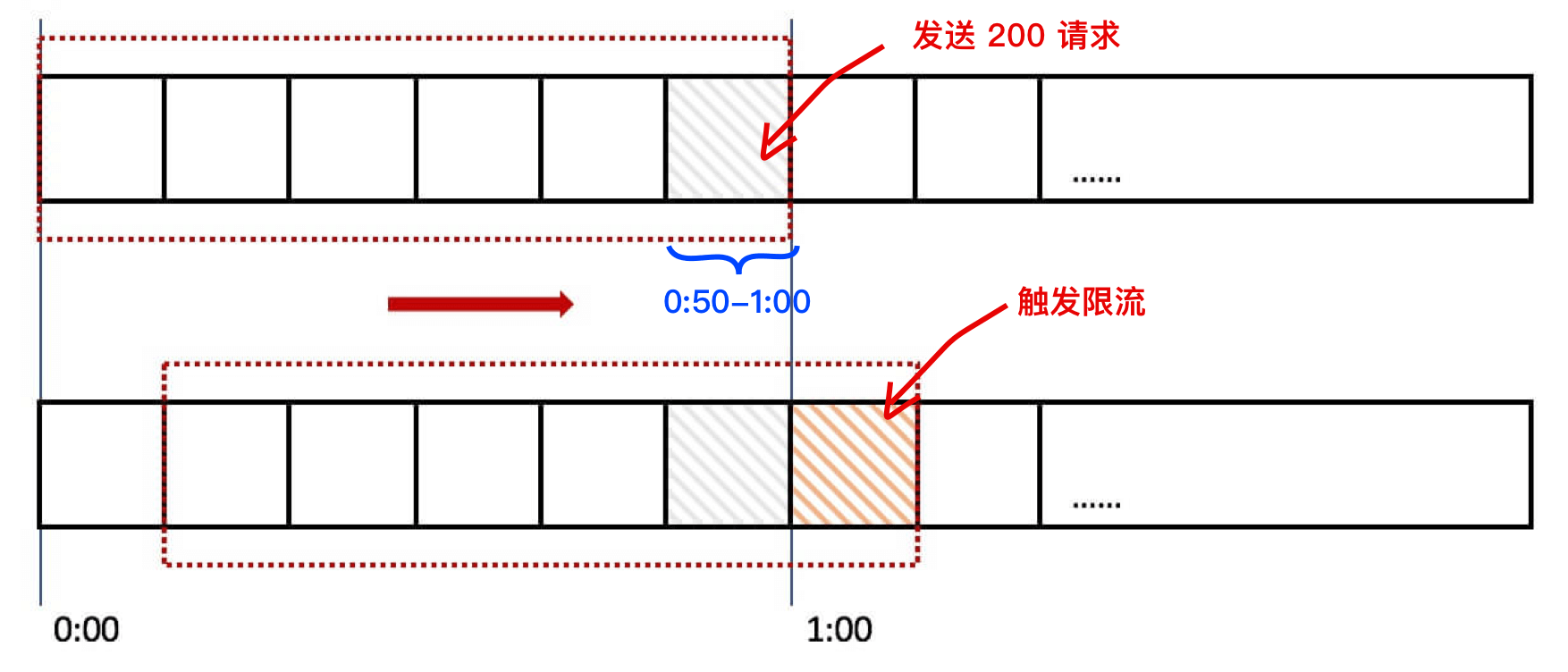### 3. 漏桶算法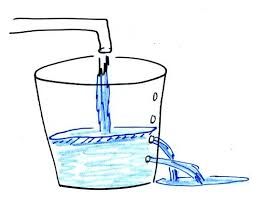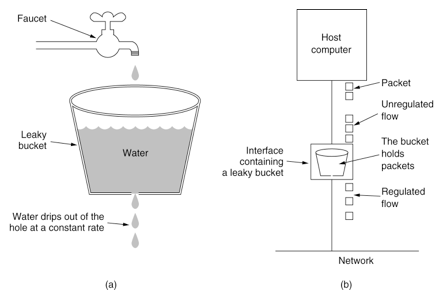``````type LeakyBucket struct {
Capacity    int64 // 桶的容量
Rate        int64 // 漏桶出水速度
Water       int64 // 当前水量(当前累积请求数)
RefreshTime int64
}

func (b *LeakyBucket) rateLimit() bool {
now := time.Now().Unix()

// 先执行漏水，计算剩余水量
b.Water = max(0, b.Water-(now-b.RefreshTime)*b.Rate);
b.RefreshTime = now

if b.Water < b.Capacity {
// 水桶还没满，继续加 1
b.Water++
return true
} else {
// 水满，拒绝流入
return false
}
}

func max(a int64, b int64) int64 {
if b > a {
return b
}
return a
}
``````

• 漏桶具有固定容量，出水速率是固定常量（流出请求）
• 如果桶是空的，则不需流出水滴
• 可以以任意速率流入水滴到漏桶（流入请求）
• 如果流入水滴超出了桶的容量，则流入的水滴溢出（新请求被拒绝）

### 4. 令牌桶算法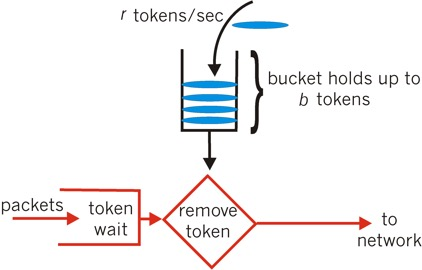``````type TokenBucket struct {
Capacity    int64 // 桶的容量
Rate        int64 // 令牌放入速度
Tokens      int64 // 当前令牌数量
RefreshTime int64
}

func (t *TokenBucket) rateLimit() bool {
now := time.Now().Unix()

// 先添加令牌
t.Tokens = min(t.Capacity, t.Tokens+(now-t.RefreshTime)*t.Rate);
t.RefreshTime = now

if t.Tokens < 1 {
// 令牌数小于 1 拒绝请求
return false
} else {
// 还有令牌，领取令牌
t.Tokens--
return true
}
}
``````

• 令牌按固定的速率被放入令牌桶中
• 桶中最多存放 B 个令牌，当桶满时，新添加的令牌被丢弃或拒绝
• 如果桶中的令牌不足 N 个，则不会删除令牌，且请求将被限流（丢弃或阻塞等待）

漏桶算法令牌桶算法